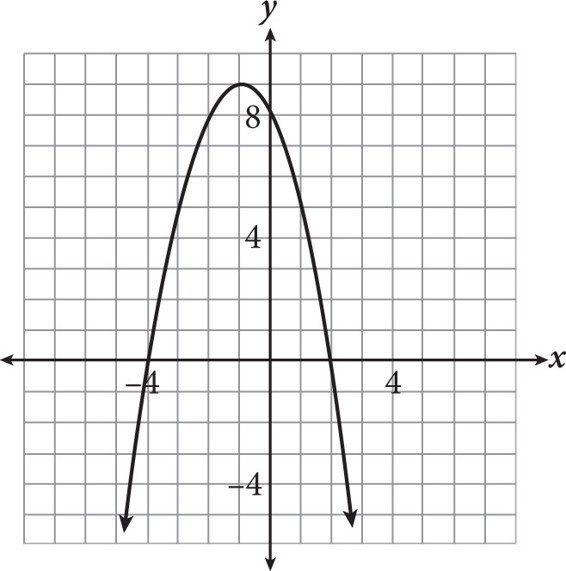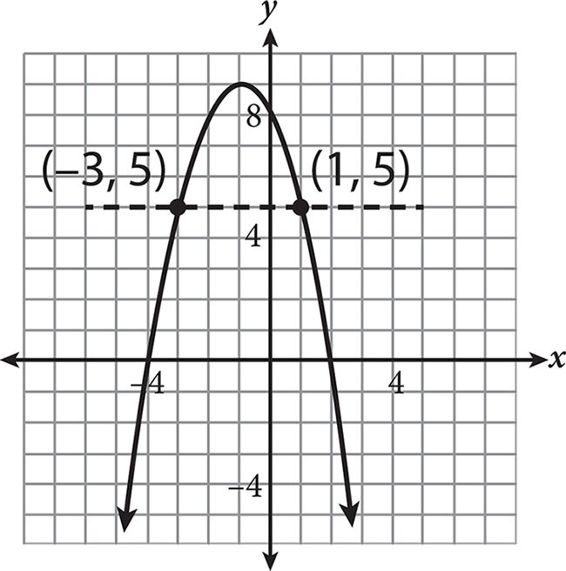# SAT Math Multiple Choice Question 469: Answer and Explanation

### Test Information

Question: 469

4.The graph of the function f(x) = –x 2 – 2x + 8 is shown in the figure above. For what values of x does f(x) = 5?

• A. –4 and 2
• B. –3 and 1
• C. –1 and 9
• D. 5 and 8

Explanation:

B

Difficulty: Medium